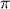# AC circuit, voltage and frequency problem

## Homework Statement

According to Equation 20.7, an ac voltage V is given as a function of time t by V = Vo sin 2ft
, where Vo is the peak voltage and f is the frequency (in hertz). For a frequency of 64.7 Hz, what is the smallest value of the time at which the voltage equals one-half of the peak-value?

## Homework Equations

V = Vo sin2(3.14)ft

## The Attempt at a Solution

1/2Vo = Vo sin(2*3.14*64.7*t)

I know I set this equation wrong with the 1/2Vo but I don't understand how to do that part, the answer I got this way is .0738 V

#### Attachments

Homework Helper
Gold Member
You need to know the angle ## \theta ## that has ##\sin(\theta)=\frac{1}{2} ##. And here, with this voltage function as a function of time, we are working in radians, so you need to write that angle ## \theta ## in radians. ## \\ ## For starters, you need to know the angle in degrees that has ## \sin(\theta)=\frac{1}{2} ##, and then convert that angle to radians.

haruspex
Homework Helper
Gold Member
I know I set this equation wrong
Looks right to me.
the answer I got this way is .0738 V

You need to know the angle ## \theta ## that has ##\sin(\theta)=\frac{1}{2} ##. And here, with this voltage function as a function of time, we are working in radians, so you need to write that angle ## \theta ## in radians. ## \\ ## For starters, you need to know the angle in degrees that has ## \sin(\theta)=\frac{1}{2} ##, and then convert that angle to radians.
How do you know its in radians?

You need to know the angle ## \theta ## that has ##\sin(\theta)=\frac{1}{2} ##. And here, with this voltage function as a function of time, we are working in radians, so you need to write that angle ## \theta ## in radians. ## \\ ## For starters, you need to know the angle in degrees that has ## \sin(\theta)=\frac{1}{2} ##, and then convert that angle to radians.
Never mind, I angular velocity=2pif

haruspex
Homework Helper
Gold Member
How do you know its in radians?
At this level, radians would be standard as input to a trig function. A calculator would generally assume radians unless you tell it otherwise.
In the present case, you have to assume that in the given sin(2ft)
the 2ft
part is in radians. The presence of the "#### Attachments

Looks right to me.

*Vo cancel out*
1/2= sin(406.5t)
1/2= sin(.523599)
t=406.5/.523599 = .0012880664 s

haruspex
Homework Helper
Gold Member
*Vo cancel out*
1/2= sin(406.5t)
1/2= sin(.523599)
t=406.5/.523599 = .0012880664 s
Correct, but don't quote so many digits. The frequency is only specified to three sig figs.

I entered .001 s and I still have it wrong

I got it, the answer was supposed to be in scientific notation